﻿ Studying Three Types of Integrals with Maple

### Studying Three Types of Integrals with Maple

Chii-Huei YuOPEN ACCESSPEER-REVIEWED

## Studying Three Types of Integrals with Maple

Department of Management and Information, Nan Jeon University of Science and Technology, Tainan City, Taiwan

### Abstract

This paper uses the mathematical software Maple for the auxiliary tool to study three types of integrals. We can obtain the infinite series forms of these three types of integrals by using integration term by term theorem. In addition, we propose some examples to do calculation practically. The research methods adopted in this study involved finding solutions through manual calculations and verifying these solutions using Maple.

• Yu, Chii-Huei. "Studying Three Types of Integrals with Maple." American Journal of Computing Research Repository 2.1 (2014): 19-21.
• Yu, C. (2014). Studying Three Types of Integrals with Maple. American Journal of Computing Research Repository, 2(1), 19-21.
• Yu, Chii-Huei. "Studying Three Types of Integrals with Maple." American Journal of Computing Research Repository 2, no. 1 (2014): 19-21.

 Import into BibTeX Import into EndNote Import into RefMan Import into RefWorks

### 1. Introduction

In calculus and engineering mathematics courses, we learnt many methods to solve the integral problems including change of variables method, integration by parts method, partial fractions method, trigonometric substitution method, and so on. In this paper, we mainly study the following three types of integrals which are not easy to obtain their answers using the methods mentioned above.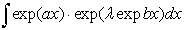(1)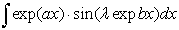(2)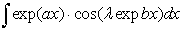(3)

where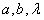are real numbers, and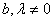. We can obtain the infinite series forms of these three types of integrals by using integration term by term theorem; these are the major results of this paper (i.e., Theorems 1-3). The study of related integral problems can refer to [1-17]. In addition, we provide some integrals to do calculation practically. The research methods adopted in this study involved finding solutions through manual calculations and verifying these solutions by using Maple. This type of research method not only allows the discovery of calculation errors, but also helps modify the original directions of thinking from manual and Maple calculations. For this reason, Maple provides insights and guidance regarding problem-solving methods.

### 2. Main Results

Firstly, we introduce some formulas used in this study.

2.1. Formulas

2.1.1. The exponential function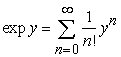, where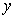is any real number.

2.1.2. The sine function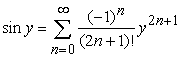, whereis any real number.

2.1.3. The cosine function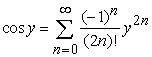, whereis any real number.

Next, we introduce an important theorem used in this paper.

2.2. Integration Term by Term Theorem ()

Suppose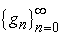is a sequence of Lebesgue integrable functions defined on an inteval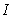. If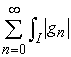is convergent, then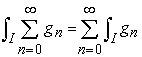.

The following is the first result of this study, we obtain the infinite series form of the integral (1).

2.3. Theorem 1

Assumeare real numbers,, and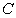is a constant. If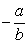is not a non-negative integer. Then the integral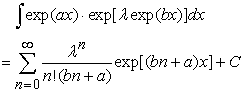(4)

Proof Because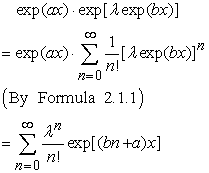(5)

It follows that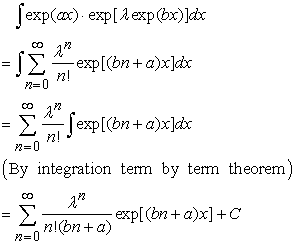Next, we determine the infinite series form of the integral (2).

2.4. Theorem 2

If the assumptions are the same as Theorem 1. If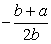is not a non-negative integer, then the integral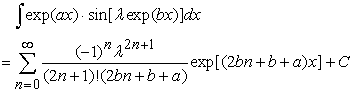(6)

Proof Because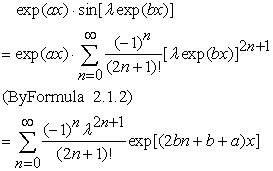(7)

We have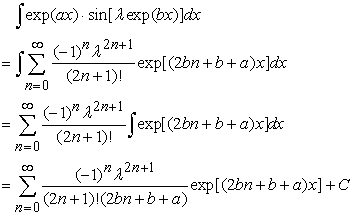The following is the third result in this study, we obtain the infinite series form of the integral (3).

2.5. Theorem 3

Let the assumptions be the same as Theorem 1. If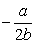is not a non-negative integer, then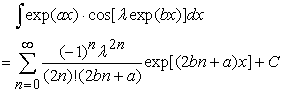(8)

Proof Because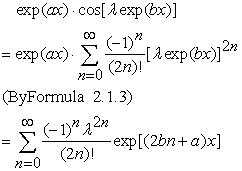(9)

It follows that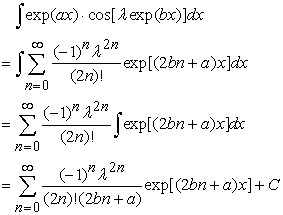### 3. Examples

In the following, for the three types of integrals in this study, we provide three integrals and use Theorems 1-3 to determine their infinite series form. On the other hand, we evaluate some related definite integrals and employ Maple to calculate the approximations of these definite integrals and their solutions for verifying our answers.

3.1. Example 1

By Theorem 1, we obtain the following integral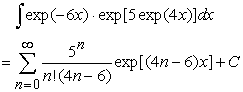(10)

Thus, we can evaluate the related definite integral from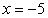to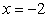,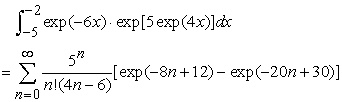(11)

We use Maple to verify the correctness of (11).

>evalf(int(exp(-6*x)*exp(5*exp(4*x)),x=-5.-2),20);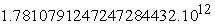>evalf(sum(5^n/(n!*(4*n-6))*(exp(-8*n+12)-exp(-20*n+30)),n=0.infinity),20);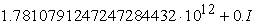The above answers obtained by Maple appears I (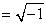), it is because Maple calculates by using special functions built in. But the imaginary part of the above answer is zero, so can be ignored.

3.2. Example 2

Using Theorem 2, we can determine the integral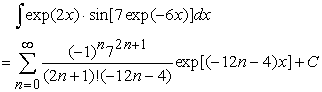(12)

Therefore, the definite integral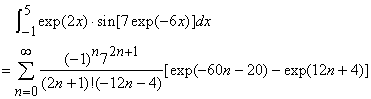(13)

>evalf(int(exp(2*x)*sin(7*exp(-6*x)),x=-1.5),24);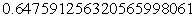>evalf(sum(((-1)^n*7^(2*n+1))/((2*n+1)!*(-12*n-4))*(exp(-60*n-20)-exp(12*n+4)),n=0.infinity),24);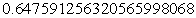3.3. Example 3

By Theorem 3, we obtain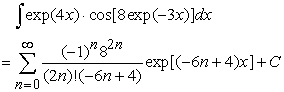(14)

Hence, the definite integral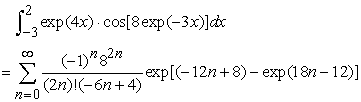(15)

>evalf(int(exp(4*x)*cos(8*exp(-3*x)),x=-3..2),18);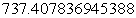>evalf(sum((-1)^n*8^(2*n)/((2*n)!*(-6*n+4))*(exp(-12*n+8)-exp(18*n-12)),n=0..infinity),18);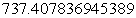### 4. Conclusion

As mentioned, the integration term by term theorem plays a significant role in the theoretical inferences of this study. In fact, the applications of this theorem are extensive, and can be used to easily solve many difficult problems; we endeavor to conduct further studies on related applications. On the other hand, Maple also plays a vital assistive role in problem-solving. In the future, we will extend the research topic to other calculus and engineering mathematics problems and solve these problems by using Maple. These results will be used as teaching materials for Maple on education and research to enhance the connotations of calculus and engineering mathematics.

### References

  M. A. Nyblom, “On the evaluation of a definite integral involving nested square root functions,” Rocky Mountain Journal of Mathematics, Vol. 37, No. 4, pp. 1301-1304, 2007.In article CrossRef  C. Oster, “Limit of a definite integral,” SIAM Review, Vol. 33, No. 1, pp. 115-116, 1991.In article CrossRef  A. A. Adams, H. Gottliebsen, S. A. Linton, and U. Martin, “Automated theorem proving in support of computer algebra: symbolic definite integration as a case study,” Proceedings of the 1999 International Symposium on Symbolic and Algebraic Computation, pp. 253-260, Vancouver, Canada, 1999.In article CrossRef  C.-H. Yu, “A study of the integrals of trigonometric functions with Maple,” Proceedings of the Institute of Industrial Engineers Asian Conference 2013, Springer, Vol. 1, pp. 603-610, 2013.In article  C.-H. Yu, “Evaluating some integrals with Maple,” International Journal of Computer Science and Mobile Computing, Vol. 2, Issue. 7, pp. 66-71, 2013.In article  C.-H. Yu, “A study of some integral problems using Maple,” Mathematics and Statistics, Vol. 2, No. 1, pp. 1-5, 2014.In article  C.-H. Yu, “Application of Maple on the integral problems,” Applied Mechanics and Materials, Vols. 479-480 (2014), pp. 849-854, 2013.In article  C.-H. Yu, “Using Maple to study two types of integrals,” International Journal of Research in Computer Applications and Robotics, Vol. 1, Issue. 4, pp. 14-22, 2013.In article  C.-H. Yu, “Using Maple to study the integrals of trigonometric functions,” Proceedings of the 6 th IEEE/International Conference on Advanced Infocomm Technology, pp. 209-215, 2013.In article  C.-H. Yu, “Solving some integrals with Maple,” International Journal of Research in Aeronautical and Mechanical Engineering, Vol. 1, Issue. 3, pp. 29-35, 2013.In article  C.-H. Yu, “Application of Maple on some type of integral problem,” Proceedings of the Ubiquitous-Home Conference 2012, pp. 206-210, 2012.In article  C.-H. Yu, “A study on integral problems by using Maple,” International Journal of Advanced Research in Computer Science and Software Engineering, Vol. 3, Issue. 7, pp. 41-46, 2013.In article  C.-H. Yu, “Application of Maple on evaluating the closed forms of two types of integrals,” Proceedings of the 17th Mobile Computing Workshop, ID 16, 2012.In article  C.-H. Yu, “Application of Maple on some integral problems,” Proceedings of the International Conference on Safety & Security Management and Engineering Technology 2012, pp. 290-294, 2012.In article  C.-H. Yu, “Application of Maple on the integral problem of some type of rational functions,” Proceedings of the Annual Meeting and Academic Conference for Association of IE, D357-D362, 2012.In article  C.-H. Yu, “Application of Maple on evaluation of definite integrals,” Applied Mechanics and Materials, Vols. 479-480 (2014), pp. 823-827, 2013.In article  C.-H. Yu, “Application of Maple: taking two special integral problems as examples,” Proceedings of the 8th International Conference on Knowledge Community, pp. 803-811, 2012.In article  T. M. Apostol, Mathematical analysis, 2nd ed., Addison-Wesley, Boston, p 269, 1975.In article